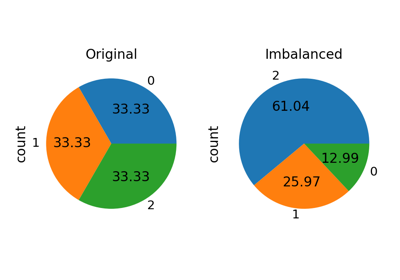# RandomUnderSampler#

class imblearn.under_sampling.RandomUnderSampler(*, sampling_strategy='auto', random_state=None, replacement=False)[source]#

Class to perform random under-sampling.

Under-sample the majority class(es) by randomly picking samples with or without replacement.

Read more in the User Guide.

Parameters
sampling_strategyfloat, str, dict, callable, default=’auto’

Sampling information to sample the data set.

• When float, it corresponds to the desired ratio of the number of samples in the minority class over the number of samples in the majority class after resampling. Therefore, the ratio is expressed aswhereis the number of samples in the minority class andis the number of samples in the majority class after resampling.

Warning

float is only available for binary classification. An error is raised for multi-class classification.

• When str, specify the class targeted by the resampling. The number of samples in the different classes will be equalized. Possible choices are:

'majority': resample only the majority class;

'not minority': resample all classes but the minority class;

'not majority': resample all classes but the majority class;

'all': resample all classes;

'auto': equivalent to 'not minority'.

• When dict, the keys correspond to the targeted classes. The values correspond to the desired number of samples for each targeted class.

• When callable, function taking y and returns a dict. The keys correspond to the targeted classes. The values correspond to the desired number of samples for each class.

random_stateint, RandomState instance, default=None

Control the randomization of the algorithm.

• If int, random_state is the seed used by the random number generator;

• If RandomState instance, random_state is the random number generator;

• If None, the random number generator is the RandomState instance used by np.random.

replacementbool, default=False

Whether the sample is with or without replacement.

Attributes
sampling_strategy_dict

Dictionary containing the information to sample the dataset. The keys corresponds to the class labels from which to sample and the values are the number of samples to sample.

sample_indices_ndarray of shape (n_new_samples,)

Indices of the samples selected.

New in version 0.4.

n_features_in_int

Number of features in the input dataset.

New in version 0.9.

NearMiss

Undersample using near-miss samples.

Notes

Supports multi-class resampling by sampling each class independently. Supports heterogeneous data as object array containing string and numeric data.

Examples

>>> from collections import Counter
>>> from sklearn.datasets import make_classification
>>> from imblearn.under_sampling import RandomUnderSampler
>>> X, y = make_classification(n_classes=2, class_sep=2,
...  weights=[0.1, 0.9], n_informative=3, n_redundant=1, flip_y=0,
... n_features=20, n_clusters_per_class=1, n_samples=1000, random_state=10)
>>> print('Original dataset shape %s' % Counter(y))
Original dataset shape Counter({1: 900, 0: 100})
>>> rus = RandomUnderSampler(random_state=42)
>>> X_res, y_res = rus.fit_resample(X, y)
>>> print('Resampled dataset shape %s' % Counter(y_res))
Resampled dataset shape Counter({0: 100, 1: 100})


Methods

 fit(X, y) Check inputs and statistics of the sampler. fit_resample(X, y) Resample the dataset. get_params([deep]) Get parameters for this estimator. set_params(**params) Set the parameters of this estimator.
fit(X, y)[source]#

Check inputs and statistics of the sampler.

You should use fit_resample in all cases.

Parameters
X{array-like, dataframe, sparse matrix} of shape (n_samples, n_features)

Data array.

yarray-like of shape (n_samples,)

Target array.

Returns
selfobject

Return the instance itself.

fit_resample(X, y)[source]#

Resample the dataset.

Parameters
X{array-like, dataframe, sparse matrix} of shape (n_samples, n_features)

Matrix containing the data which have to be sampled.

yarray-like of shape (n_samples,)

Corresponding label for each sample in X.

Returns
X_resampled{array-like, dataframe, sparse matrix} of shape (n_samples_new, n_features)

The array containing the resampled data.

y_resampledarray-like of shape (n_samples_new,)

The corresponding label of X_resampled.

get_params(deep=True)[source]#

Get parameters for this estimator.

Parameters
deepbool, default=True

If True, will return the parameters for this estimator and contained subobjects that are estimators.

Returns
paramsdict

Parameter names mapped to their values.

set_params(**params)[source]#

Set the parameters of this estimator.

The method works on simple estimators as well as on nested objects (such as Pipeline). The latter have parameters of the form <component>__<parameter> so that it’s possible to update each component of a nested object.

Parameters
**paramsdict

Estimator parameters.

Returns
selfestimator instance

Estimator instance.

## Examples using imblearn.under_sampling.RandomUnderSampler#How to use sampling_strategy in imbalanced-learn#Example of topic classification in text documents#Fitting model on imbalanced datasets and how to fight bias#